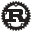Rust 1.62.0
a8314ef7d

# Casting between types

There is a new edition of the book and this is an old link.

A type cast expression is denoted with the binary operator `as`. Executing an `as` expression casts the value on the left-hand side to the type on the right-hand side.

``````
fn average(values: &[f64]) -> f64 {
let sum: f64 = sum(values);
let size: f64 = len(values) as f64;
sum / size
}``````
Run

Here are the relevant sections in the new and old books: